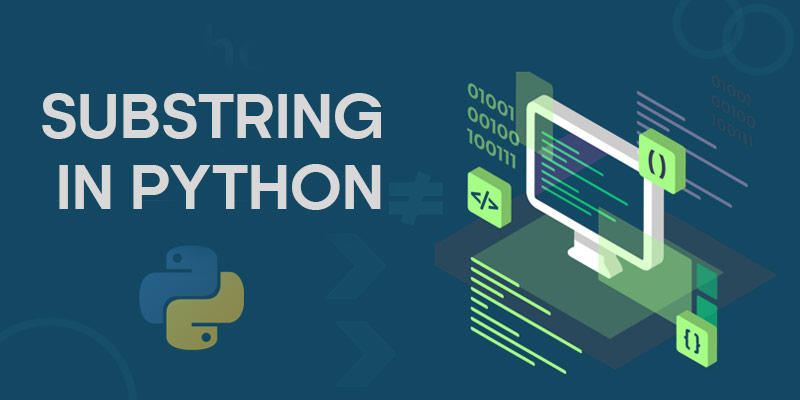We know that a string is a sequence of characters, but today we will learn how to get a part of any string, known as substring.

Checkout this complete Online Python Training by FITA. FITA provides a complete Python course where you will be building real-time projects like Bitly and Twitter bundled with Django, placement support and certification at an affordable price.

### String Slicing

String slicing can be done using the [] (square brackets) notation, just as you get index.

Slice for a character

The string[index] will return a single character of your string at the index.Since the indexing starts at 0, the string will give you the very first character and the string[-1] will give the very last character.And the rest of the characters can be found using their position

 my_string = ‘Get a complete Python tutorial at FITA’ print(my_string) print(my_string[len(my_string)//2]) print(my_string[-1])

And the above code will output..

 G o A

You can get the training even if you are not in GOA.

Slicing characters starting at n.

The string[first_index:] will give you all the characters starting from the index given.

 print(my_string[6:]) print(my_string[6::])

This will give give you all the characters except for the first 6,both the lines will give you the same output as

 complete Python tutorial At FITA
Slicing characters for first n characters.

The string[:end_index] will give all the characters until the given index.

 my_string =’Get a complete Python tutorial At FITA’ print(my_string[:6])

This will give you the first 6 characters of the my_string.

 Get a
Slicing characters with a jump or step.

The string[::step] will give all the characters with a step or skip of the the value

For example

 my_string =’Get a complete Python tutorial At FITA’ print(my_string[::11])

This will give you characters starting from 0 till the end but with a skip of 11 characters in between.Here is the output..

 Get

Note that this is not the first 3 characters, let me give another example

 print(my_string[::25])

Output

 Go

Now let’s fill all the gaps with a start ,end, step.

Slice characters from start to end with a step.

The my_string[start:end:step] will give you the characters from starting position to the end position with a skip of step characters.

 my_string =’Get a complete Python tutorial At FITA’ print(my_string[4:35:12]) print(my_string[9::4]) print(my_string[:24:10]) print(my_string[9:18:4])

Here is the output for the above

 aya pet ol A Gln pet
Negative indexing

A negative index will mean that you start from the end i.e., from right to left.

 print(my_string[-2]) # T

will give the last second character.

 print(my_string[-6:]) # t FITA

will give last 6 characters

 print(my_string[6:-6]) # complete Python tutorial

Will give all characters except the first 6 and last 6 characters.

### find() method

The find() is a built-in method in python which will return the starting index of the substring in the string, else return -1 if it’s not present. For instance,

 s = ‘Get a complete Python tutorial At FITA’ print(s.find(‘FITA’))

Will give you 34.

### Check for a substring

You can also use the in or not in keywords to check if a substring is present in the string, which will give a boolean result.

 my_string = ‘Get a complete Python tutorial At FITA’ print(‘ATUFA’ not in my_string) print(‘complete’ in my_string)

Which outputs..

 True True

Also you can count the number of occurrences of the substring , when you have checked if it is present, else it will return 0.

 my_string = ‘Get a complete Python tutorial At FITA’ print(my_string.count(‘FIT’)) print(my_string.count(‘a’))

Output

 1 4

### Splitting Strings

You can split a string at a particular substring and simultaneously create a list of all the remaining substrings excluding split substring using the .split() method.

Here’s an example of the split method.

 my_string = ‘Get a complete Python tutorial At FITA’ print(my_string.split(‘ ‘)) print(my_string.split(‘P’))

In the first print statement I am splitting at space, and in the second one at P.

Here’s the output

 [‘Get’, ‘a’, ‘complete’, ‘Python’, ‘tutorial’, ‘At’, ‘FITA’] [‘Get a complete ‘, ‘ython tutorial At FITA’]

You can now even join these substrings using the .join() method.

This was all about slicing strings in python with its implementation..To get in-depth knowledge of Python along with its various applications and real-time projects, you can enroll in Python Training in Chennai or Python Training in Bangalore by FITA at an affordable price, which includes real time projects with certification, support and career guidance assistance.

﻿